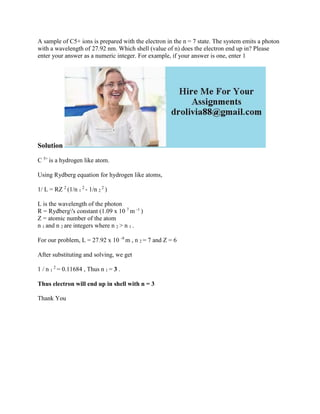Successfully reported this slideshow.

# A sample of C5+ ions is prepared with the electron in the n - 7 state-.docx×

# A sample of C5+ ions is prepared with the electron in the n - 7 state-.docx

A sample of C5+ ions is prepared with the electron in the n = 7 state. The system emits a photon with a wavelength of 27.92 nm. Which shell (value of n) does the electron end up in? Please enter your answer as a numeric integer. For example, if your answer is one, enter 1
Solution
C 5+ is a hydrogen like atom.
Using Rydberg equation for hydrogen like atoms,
1/ L = RZ 2 (1/n 1 2 - 1/n 2 2 )
L is the wavelength of the photon
R = Rydberg\'s constant (1.09 x 10 7 m -1 )
Z = atomic number of the atom
n 1 and n 2 are integers where n 2 > n 1 .
For our problem, L = 27.92 x 10 -9 m , n 2 = 7 and Z = 6
After substituting and solving, we get
1 / n 1 2 = 0.11684 , Thus n 1 = 3 .
Thus electron will end up in shell with n = 3
Thank You
.

A sample of C5+ ions is prepared with the electron in the n = 7 state. The system emits a photon with a wavelength of 27.92 nm. Which shell (value of n) does the electron end up in? Please enter your answer as a numeric integer. For example, if your answer is one, enter 1
Solution
C 5+ is a hydrogen like atom.
Using Rydberg equation for hydrogen like atoms,
1/ L = RZ 2 (1/n 1 2 - 1/n 2 2 )
L is the wavelength of the photon
R = Rydberg\'s constant (1.09 x 10 7 m -1 )
Z = atomic number of the atom
n 1 and n 2 are integers where n 2 > n 1 .
For our problem, L = 27.92 x 10 -9 m , n 2 = 7 and Z = 6
After substituting and solving, we get
1 / n 1 2 = 0.11684 , Thus n 1 = 3 .
Thus electron will end up in shell with n = 3
Thank You
.